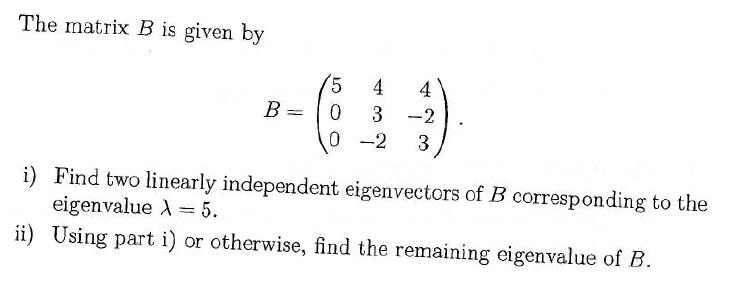# Find 2 eigenvectors given an eigenvalue, and find remaining eigenvalueit looks easy but i forgot how to do it -__-

i did A - lamda I = 0, substituted lamda = 5, then my matrix became

0 4 4
0 -2 -2
0 -2 -2

so i put in the form of A|b where b was a zero vector and i row reduced, getting
0 1 1 | 0
0 0 0 | 0
0 0 0 | 0

and now i dont know what to do.

edit: there have been some silly errors to other solutions for this particular past paper, like a missing - sign. just a heads up in case the answer is a tad wrong.

Last edited:

Mark44
Mentorit looks easy but i forgot how to do it -__-

i did A - lamda I = 0, substituted lamda = 5, then my matrix became

0 4 4
0 -2 -2
0 -2 -2

so i put in the form of A|b where b was a zero vector and i row reduced, getting
0 1 1 | 0
0 0 0 | 0
0 0 0 | 0

and now i dont know what to do.
This is a system of one equation in three variables, so there are two variables that are free.

The nonzero row represents the equation x2 + x3 = 0.
To get all three variables involved, we can write
x1 = x1
x2 = -x3
x3 = x3

So any vector x that is an eigenvector for the eigenvalue 5 is a linear combination of two vectors. Can you see them here?

edit: there have been some silly errors to other solutions for this particular past paper, like a missing - sign. just a heads up in case the answer is a tad wrong.

Last edited:
i sort of get it...

what do you mean when you say x3 = x ?

the worked solutions that i have say eigenvectors are:

(x1,x2,x3) = (x1,-x3,x3)
which makes sense to me

but then they split it up:
(x1,x2,x3) = (x1,-x3,x3) = (x1,0,0) + (0,-x3,x3)

then take x1 and x3 out the front as arbitrary non-zero constants (which i get).

Why do they split up (x1,-x3,x3), is it only because the question is asking for 2 eigenvectors?
and in that case can you just split it up any way so that when you add them they still equal to (x1,-x3,x3)?

Mark44
Mentor
i sort of get it...

what do you mean when you say x3 = x ?
That should have said x3 = x3. I neglected to get the subscript in between my [ sub] tags. I fixed it in my earlier post.
the worked solutions that i have say eigenvectors are:

(x1,x2,x3) = (x1,-x3,x3)
which makes sense to me

but then they split it up:
(x1,x2,x3) = (x1,-x3,x3) = (x1,0,0) + (0,-x3,x3)

then take x1 and x3 out the front as arbitrary non-zero constants (which i get).

Why do they split up (x1,-x3,x3), is it only because the question is asking for 2 eigenvectors?
and in that case can you just split it up any way so that when you add them they still equal to (x1,-x3,x3)?

They split <x1, -x3, x3> to get two vectors.
<x1, -x3, x3> = x1<1, 0, 0> + x3<0, -1, 1>. Since x1 and x3 are arbitrary, this represents all linear combinations of <1, 0, 0> and <0, -1, 1>.

(All vectors are column vectors here.>

ohhhhhhhhh i get it now, they split it up into the 2 vectors, one vector includes only the x1 component and the other one only includes the x3 components.

thanks a lot :)

Mark44
Mentor
Keep that trick in mind! It comes in handy in a lot of problems...

HallsofIvy
"$\lambda$ is an eigenvalue of A if and only if there exist a non-zero vector v such that $Av= \lambda v$".